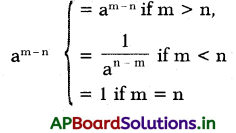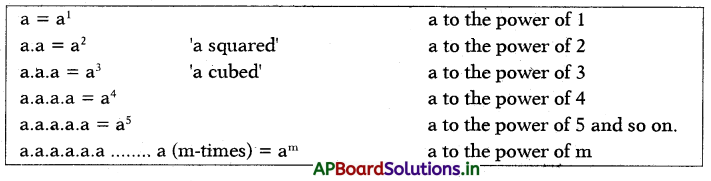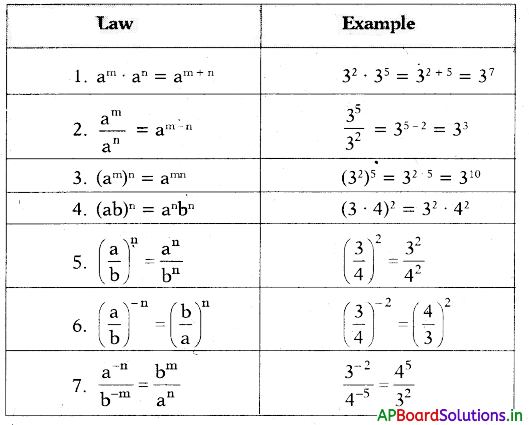# AP 7th Class Maths Notes 8th Lesson Exponents and Powers

Students can go through AP Board 7th Class Maths Notes 8th Lesson Exponents and Powers to understand and remember the concept easily.

## AP Board 7th Class Maths Notes 8th Lesson Exponents and Powers

→ To make large numbers easy to read, write and understand in a simple man¬ner exponents help us in doing so
Ex: 2 × 2 × 2 × 2× 2 × 2 = 26
a × a × a … ‘n’ times = an

→ Laws of exponents :
For any non-zero integers ‘a’ and ‘b’ and integers ‘m’ and ‘n

• am × an = am+n
• (am)n = amn• am × bm = (ab)m
• am ÷ bm = $$\left(\frac{a}{b}\right)^{m}$$
• a0 = 1 (where a ≠ 0)
• (-1)even number = 1
• (-1 )odd number = -1

→ In standard form a number is expressed as the product of largest integer exponent of 10 and any decimal number from 1 to 9.

→ Repeated addition can be expressed as multiplication i.e., if a number ‘a’ is added to itself for n-times we write it as na.
a + a + a + a + ………………… a (n-times) = n.a = na→ If a number a is multiplied by itself n- number of times, We can express in exponential form.
a . a . a . a . a . a . a . a . a ……………….. (n-times) = an. This is read as a to the power of n. Here a is called base and n is called exponent.

→ Exponential Form:
Example:→ A natural number can be expressed in exponential form using its prime factors. Write the given number as product of primes and the express in, exponential form.
Example : 72 = 2 × 2 × 2 × 3 × 3 = 23 × 32
405 = 3 × 3 × 3 × 3 × 5 = 34 × 5

→ Laws of Exponents:

 Zero Exponent a0 = 1 a ≠ 0 Any value to the power of £) equals 1 , unless the value is 0 Exponent of one a1 = a Any value to the power of 1 is itself Negative exponent a-m = 1/am Inverse a-1 = 1/a Product am × an = a(m+n) If both bases are the same, add the exponents Quotient am/an = a(m-n) If both bases are the same, subtract the exponents Power of a power (am)n = amn Power of a product ambm = (ab)m→ Standard form: In standard form, a number is expressed as a product of integer exponent of 10 and a decimal number from 1 to 9.
Example: 8569 = 8.569 × 1000 = 8.569 × 103